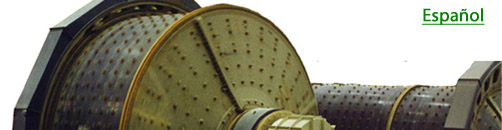expertise in mineral grinding systemsIssue No. 6                                                                                     February, 2015   Calculate the 'Work Index Efficiency' of your grinding circuit.   Historically (before Bond), in order to be able to size ball mills for new projects, Allis-Chalmers ran standard laboratory grindability tests on samples of the ore from numerous plant circuits. The 'grams per revolution' ground through a given screen size (e.g. 75 um) measured on the ore was correlated to the kWh per net ton of similar product size material being produced by the plant circuit. For a new project, a sample of the new ore was subjected to the same grindability test. Then, by comparison with their test database, the required kWh per ton for the new mill could be estimated, and along with the design tonnage, the needed mill size could be determined.  Armed with his new "Work Index" equation, Bond converted both the grindability test data and corresponding plant data into "Work Index" values. While dealing with scatter in the data, he found that they could be mathematically correlated. Then, by applying a 'scale-up' factor to the Laboratory Work Index, he made it, on average, equal to the Operating Work Index. The lab test was renamed the now familiar "Bond Work Index Test", and the new mill sizing method was broadly adopted.  Given that the newly formulated "Bond Test Work Index" prescribed the expected circuit Operating Work Index, comparison of the two yielded a relative circuit efficiency value. For design, the Test Work Index (Wlt) equals the Operating Work Index (WIo), all tied to the average energy efficiency of the circuits in the data base. For any given circuit, when Wlo < Wlt, the circuit is more efficient than that average, and when Wlo > Wlt, less efficient. The ratio between them thus quantitatively expresses relative "Circuit Bond Work index Efficiency".  We showed you how to calculate your grinding circuit's Operating Work Index in Grinding Bulletin No. 5: "What exactly is "Work Index" and how can I make use of it?" In this basic example, the Operating Work Index was calculated to be 14.4 kWh/t. The Bond Ball Mill Test Work Index of the ore was measured to be 15.8 kWh/t. Then, taking Work Index Efficiency as the ratio of Test WI / Operating WI, it equals 15.8 / 14.4 = 1.10, or 110%. This circuit is approximately 10% more efficient than the average circuit upon which the Bond design method is based. Alternatively, take the ratio 14.4 / 15.8 = 0.91. The circuit is using approximately 91% of the prescribed Bond energy.   By calculating your circuit's Work Index Efficiency, you can compare it to the Bond average of 100%. Thanks to the amazing standardization of size reduction that the concept of "Work Index" provides, you can also compare it to the efficiency of other circuits, both similar and dissimilar. And you can monitor efficiency increases as you work through a grinding circuit efficiency improvement program. References: "Determination of Ball Mill Size from Grindability Data", S.D. Michaelson, AIME Trans. V. 169, 1946, pp. 123-128. "Using the Bond Work Index to Measure Operating Comminution Efficiency", C.A. Rowland, Jr., Minerals and Metallurgical Processing, V. 15, No. 4, November, 1998, pp. 32-36.